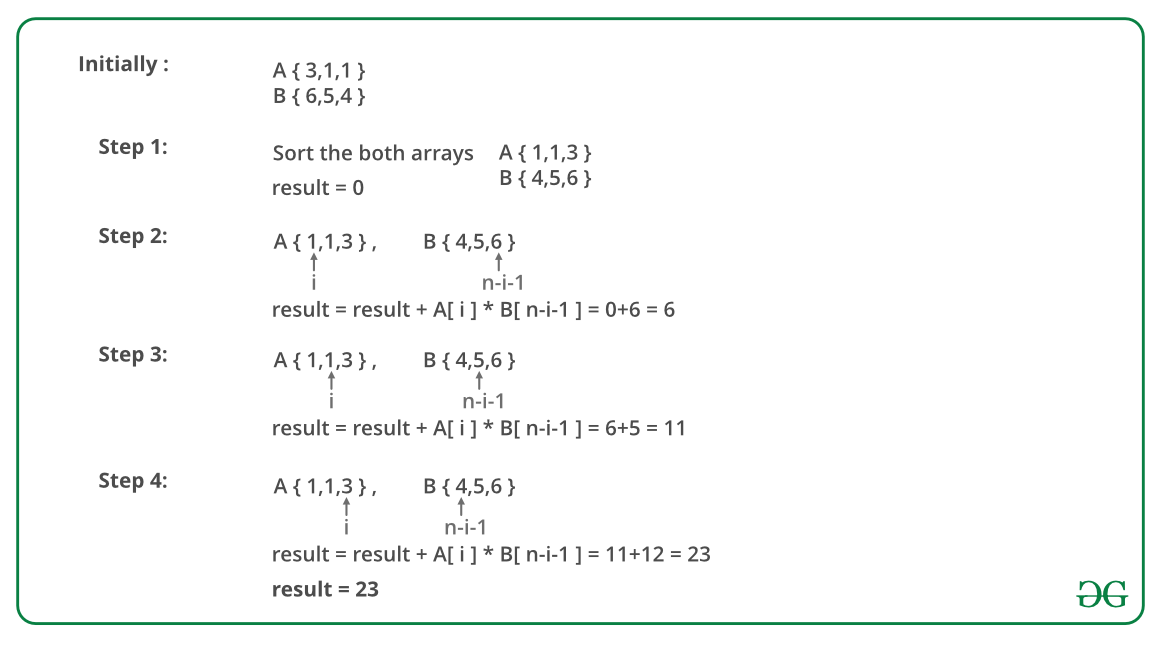Minimize the sum of product of two arrays with permutations allowed

• Difficulty Level : Easy
• Last Updated : 25 Mar, 2021

Given two arrays, A and B, of equal size n, the task is to find the minimum value of A * B + A * B +…+ A[n-1] * B[n-1]. Shuffling of elements of arrays A and B is allowed.

Examples :

Input : A[] = {3, 1, 1} and B[] = {6, 5, 4}.
Output : 23
Minimum value of S = 1*6 + 1*5 + 3*4 = 23.

Input : A[] = { 6, 1, 9, 5, 4 } and B[] = { 3, 4, 8, 2, 4 }
Output : 80.
Minimum value of S = 1*8 + 4*4 + 5*4 + 6*3 + 9*2 = 80.

Recommended: Please solve it on “PRACTICE” first, before moving on to the solution.

The idea is to multiply minimum element of one array to maximum element of another array. Algorithm to solve this problem:

1. Sort both the arrays A and B.
2. Traverse the array and for each element, multiply A[i] and B[n – i – 1] and add to the total.

Note: We are adding multiplication of elements which can lead to overflow conditions.

Below image is an illustration of the above approach:Below is the implementation of the above approach:

C++

 // C++ program to calculate minimum sum of product// of two arrays.#include using namespace std;   // Returns minimum sum of product of two arrays// with permutations allowedlong long int minValue(int A[], int B[], int n){    // Sort A and B so that minimum and maximum    // value can easily be fetched.    sort(A, A + n);    sort(B, B + n);       // Multiplying minimum value of A and maximum    // value of B    long long int result = 0;    for (int i = 0; i < n; i++)        result += (A[i] * B[n - i - 1]);       return result;}   // Driven Codeint main(){    int A[] = { 3, 1, 1 };    int B[] = { 6, 5, 4 };    int n = sizeof(A) / sizeof(A);    cout << minValue(A, B, n) << endl;    return 0;}

Java

 // Java program to calculate minimum// sum of product of two arrays.import java.io.*;import java.util.*; class GFG {     // Returns minimum sum of product of two arrays    // with permutations allowed    static long minValue(int A[], int B[], int n)    {        // Sort A and B so that minimum and maximum        // value can easily be fetched.        Arrays.sort(A);        Arrays.sort(B);         // Multiplying minimum value of A        // and maximum value of B        long result = 0;        for (int i = 0; i < n; i++)            result += (A[i] * B[n - i - 1]);         return result;    }     // Driven Code    public static void main(String[] args)    {        int A[] = { 3, 1, 1 };        int B[] = { 6, 5, 4 };        int n = A.length;        ;        System.out.println(minValue(A, B, n));    }} // This code is contributed by vt_m

Python

 # Python program to calculate minimum sum of product# of two arrays. # Returns minimum sum of product of two arrays# with permutations allowed  def minValue(A, B, n):     # Sort A and B so that minimum and maximum    # value can easily be fetched.    A.sort()    B.sort()     # Multiplying minimum value of A and maximum    # value of B    result = 0    for i in range(n):        result += (A[i] * B[n - i - 1])     return result  # Driven ProgramA = [3, 1, 1]B = [6, 5, 4]n = len(A)print minValue(A, B, n) # Contributed by: Afzal Ansari

C#

 // C# program to calculate minimum// sum of product of two arrays.using System; class GFG {     // Returns minimum sum of product    // of two arrays with permutations    // allowed    static long minValue(int[] a, int[] b,                                   int n)    {                 // Sort A and B so that minimum        // and maximum value can easily        // be fetched.        Array.Sort(a);        Array.Sort(b);         // Multiplying minimum value of        // A and maximum value of B        long result = 0;                 for (int i = 0; i < n; i++)            result += (a[i] * b[n - i - 1]);         return result;    }     // Driven Code    public static void Main()    {                 int[] a = { 3, 1, 1 };        int[] b = { 6, 5, 4 };        int n = a.Length;                 Console.Write(minValue(a, b, n));    }} // This code is contributed by nitin mittal.



Javascript



Output :

23

Time Complexity : O(n log n).

This article is contributed by Anuj Chauhan(anuj0503). If you like GeeksforGeeks and would like to contribute, you can also write an article using write.geeksforgeeks.org or mail your article to review-team@geeksforgeeks.org. See your article appearing on the GeeksforGeeks main page and help other Geeks.